# Bernstein polynomials

Algebraic polynomials defined by the formula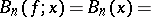Introduced by S.N. Bernshtein in 1912 (cf. ). The sequence of Bernstein polynomials converges uniformly to a functionon the segment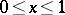ifis continuous on this segment. For a function which is bounded by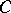,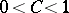, with a discontinuity of the first kind,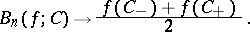The equationis valid ifis twice differentiable at the point. If the-th derivative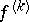of the function is continuous on the segment, the convergence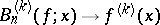is uniform on this segment. A study was made ([1b], ) of the convergence of Bernstein polynomials in the complex plane ifis analytic on the segment.